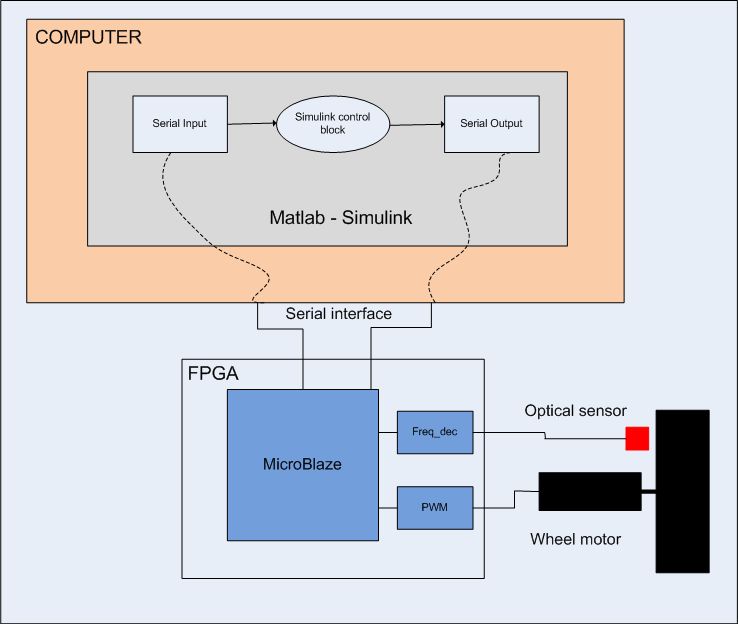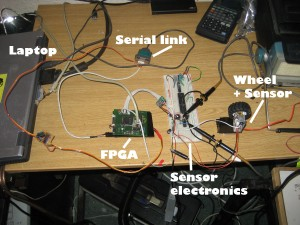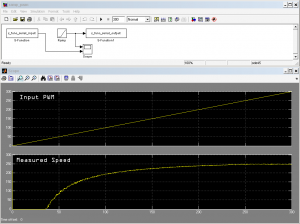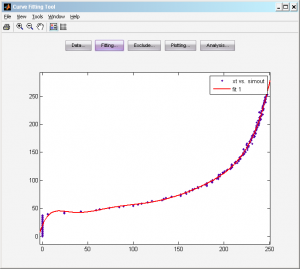# Testing realtime PID in Simulink on HardwareThis information was contained in my Quatbot project post, but I have moved it here instead.

I decided to test out some PID control of the speed of a wheel in a project I was making. I thought it might be interesting to interface directly with Simulink in Matlab and try and use the provided Toolbox.

This allowed the ability to capture data, control speed, and used the Simulink control block ‘live’ in real time with the hardware.

So in order to do this I connect the motor and optical sensor to an FPGA, the FPGA then connected via a serial link to the computer.

Below show the setup used:Simulink allows the creation of custom blocks called S-functions. I created 2 S-functions, one for reading serial data – this acted as a Simulink source; and the other which wrote serial data – this acted as a Simulink sink.

These 2 files are here:

s_func_serial_input.m

s_func_serial_output.m

A picture of the hardware is show below:Wheel speed VS PWM duty

Below shows actual wheel speed vs ramped input PWM duty. The flat bit at the start is a programmed dead zone, this was to try and make it slightly more linear. But as you can see, it is still very non-linear!Wheel speed vs ramped input

In order to make the wheel speed linear w.r.t PWM duty, I created a lookup table in Matlab as follows:

• Captured measured speed to Matlab array
• Reversed X and Y on captured data
• Used reversed data in Matlab Curve Fitting Tool (cftool)
• Created polynomial of curve (used 9th order)
• Generate Lookup table from polynomial functionThe same test as above was performed again, but this time with the lookup table inserted after the input data. As you can see the wheel speed is now much more linear. This is useful when using any control loop.PID Control

Using the standard PID control block in Simulink, I connected up the closed loop system. The output of the PID is sent though the Lookup Table created above. The desired speed (Input Speed) was defined as an array in Matlab.

Below shows the Simulink diagram, and the results after a run:

Input Speed – The desired speed of the wheel

Output Speed – The actual measure speed of the wheel

PID Output – The PWM duty being sent to the motor

Error Signal – The difference between Input Speed and Output Speed

The update rate was about 50ms. The real time delays in the system are:

Laptop CPU (low), Serial transmission(medium), USB->Serial latency(high).

When running, the simulation will simply run as fast as possible, and has no real concept about time. In fact Simulink doesn’t even know it is running with a real system in real time.Below shows a run when the PID parameters are incorrectly set, rendering it unstable.